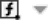If you select a range of cells, MyOffice Spreadsheet automatically calculates the most commonly used functions and displays the results in the Status bar:

SUM: Calculates the sum of all numbers in the selected range.

AVERAGE: Calculates the average value of all numbers in the selected range.

MIN: Displays the smallest value among all the numbers in the selected range.

MAX: Displays the highest value among all the numbers in the selected range.

COUNTA: Displays the number of values in the selected range. Numerical and text values are considered.

COUNT: Displays the number of numeric values in the selected range.

To configure the list of available functions, follow these steps:

1. In the Status bar, clickFunctions in Status Bar.

2. In the opened list, check the boxes of the functions that will be displayed in the Status bar.

Was the material useful?
Yes
No
Users found this material useful: 0 из 0